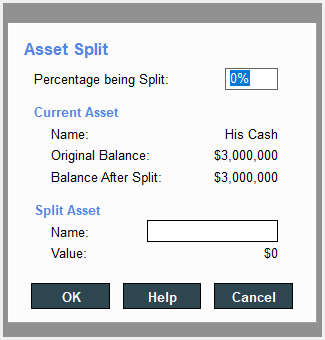Tagged:

• Author
Posts
•The Kugler Estate Analyzer assumes that each Technique uses 100% of the asset funding it. However, it is not uncommon to fund a technique with only a portion of a given asset.

Press the Split Asset button to split an existing asset into two portions. Splitting an asset creates two identical assets (you’ll have to give the new piece a new name), each of which is a percentage of the original asset. Once it has been split, the new asset can be used by any technique.

For example, if you wanted to take a portion of an asset and put it in a GRAT, you would split the asset by the percentage you want to move.

Splitting the asset may be the only way to fund certain techniques.

To split the asset, click the Split Asset button. The Split Asset window appears. Assign a percentage for the split. The window lists the asset’s original name, the original balance, and the balance after the split takes place. Once you split the asset, give it a new name. The program calculates its value.